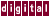# 7.4 Loop Unrolling

Loop unrolling is a standard manual optimization that creates larger loops by replication of the original loop body. Loop unrolling is done automatically by KAP to speed up some loops by reducing the number of times the loop control overhead is encountered. Inner loop unrolling is controlled by the `/unroll` and `/unroll2` qualifiers. Outer loop unrolling is part of memory management and is controlled by the `/roundoff` and `/scalaropt` qualifiers.

Unrolling a loop involves duplicating the loop body one or more times within the loop, adding an increment, or changing the increment that was already in the loop, and possibly inserting cleanup code before the loop to execute any left-over iterations of the loop. If the loop bounds are constant and the iteration count of the loop is small, the loop may be entirely deleted and replaced by copies of the loop body.

If the loop bounds are constant, KAP may use an unrolling factor near, but above, the unroll value if that will exactly divide the loop iteration count.

The `/scalaropt` command qualifier must be set to at least 2 to enable loop unrolling.

The following examples were run with `/unroll=8` and `/unroll2=1000` . See Chapter 4 for more information about these command qualifiers.

If the loop bounds are unknown at compilation time, a loop may be unrolled, as shown in the following example:

```for (i=1; i<n ; i++)
a[i] = b[i]/a[i-1] ;
```

Becomes:

```for ( i = 1; i<=n - 8; i+=8 ) {
a[i] = b[i] / a[i-1];
a[i+1] = b[i+1] / a[i];
a[i+2] = b[i+2] / a[i+1];
a[i+3] = b[i+3] / a[i+2];
a[i+4] = b[i+4] / a[i+3];
a[i+5] = b[i+5] / a[i+4];
a[i+6] = b[i+6] / a[i+5];
a[i+7] = b[i+7] / a[i+6];
}
for ( ; i<n; i++ ) {
a[i] = b[i] / a[i-1];
}
```

If loop bounds are constant, the unrolled loop may look like the following example. Notice that KAP has deviated slightly from the unroll value to make the iteration count an exact multiple of the unrolling factor thereby eliminating the need for a cleanup loop, as shown in the following example:

```for (i=1; i<100; i++)
a[i] = b[i]/a[i-1] ;
```
Becomes:
```for ( i = 1; i<=91; i+=9 ) {
a[i] = b[i] / a[i-1];
a[i+1] = b[i+1] / a[i];
a[i+2] = b[i+2] / a[i+1];
a[i+3] = b[i+3] / a[i+2];
a[i+4] = b[i+4] / a[i+3];
a[i+5] = b[i+5] / a[i+4];
a[i+6] = b[i+6] / a[i+5];
a[i+7] = b[i+7] / a[i+6];
a[i+8] = b[i+8] / a[i+7];
}
```

Or, if the loop iteration count is constant and small, the loop control may be removed altogether, as shown in the following example:

```for (i=1; i<5 ; i++)
a[i] = b[i]/a[i-1] ;
```

Becomes:

```   a = b / a;
a = b / a;
a = b / a;
a = b / a;
```

Previous Page Next Page Contents Index
Command-Line Qualifiers